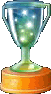ResultsTutorialAccountForums

## A Parent’s Survival Guide To Physics:  Topic One: Measuring Length

Place: 12th place in Nonfiction

A Parent’s Survival Guide To Physics:

Topic One:
Measuring Length: (inches - in, feet - ft, yards - yd, centimeters – cm, and meters – m)
by Me’ira Pitkapaasi

Measurement in Physics is not quite as simple as taking out your measuring tape.  This tool will come in handy occasionally, such as in this passage, and when you’re determining lengths that are large enough to see and short enough to personally reach.  On our measuring tape, that would be 1/8th of an inch to a yard; or a millimeter to a meter.  In the greater scientific community, we use the metric system, so in order to understand what your student is learning, you’re going to finally have to figure out how this system works!

An inch is typically the smallest unit of length used by the average parent in the U.S.A., or a fraction thereof.  We were always taught that an inch is about two and a half centimeters, which was correct!  So how do we compute length from there?  We use a formula that you can understand if you can compute multiplication and division problems with your calculator, and you can remember that 2 ½ = 2.5 in your calculator.

_____ centimeters = (2.5 centimeters) x (however many inches you just measured) ÷ (1 inch)
Or
______ = 2.5 x (measured number) ÷ 1
Or
_______ = 2.5 x (measured number) because any number ÷ 1 stays the same.

So if you’re measuring length of a standard piece of copy paper, you’re going to find that it’s 11 inches long.
________ = 2.5 x 11
= 27.5 centimeters
Pretty cool, eh?

What if we’re measuring feet though? There are 30.5 centimeters in a foot.

______ centimeters = (30.5 centimeters) x (however many feet you just measured) ÷ (1 foot)
Or
______ = 30.5 x (measured number of feet) ÷ 1
Or
_______ = 30.5 x (measured number)

If you measured how tall your living room is, you might have discovered it was 7 feet tall.  Let’s put it into our equation:

______ = 30.5 x (7)
= 214 centimeters.

Now there has to be a simpler way to say “My living room is 214 centimeters tall.”
There is.  You must remember that there every 100 centimeters = 1 meter.  So your living room would be 2 meters (for the 200 centimeters) with 14 centimeters left over.  And the metric system gives us a pretty easy way to add those 14 centimeters to our 2 meters.  It’s very different than how we use feet and inches. Here’s a new formula for you:

_____ meters total = (number of whole meters) “.” (number of centimeters left over).
_____ = 2 . 14
_____ = 2.14 meters

Football fields are a bit long for your tape measure, but we know they’re 100 yards long.  Knowing there are 214 centimeters in the height of your living room might prove to you that using centimeters to measure a football field isn’t the greatest method of metric length measurement.

Let’s move on to the meter.  Just as we were often told an inch is around 2 ½ centimeters, we were often told that a meter was “a little more than a yard.”  Once again, fairly accurate. One meter = 1.1 years.  So how do we find the length of a football field?  Here it gets a little more difficult than above.  After the division sign, we have a number that is not one, so it cannot drop out of our formula.

______ meters = (1 meter) x (number of yards measured)  ÷ (1.1 yards)
______ meters = 1 x (number of yards measured) ÷ 1.1
(1 x any number stays the same, so the 1x can drop out)
______ meters = (number of yards measured) ÷ 1.1
______ meters = 100 ÷ 1.1
= 110 meters

You can now measure the basics of length with your student!

Next passage:
Lengths both Tiny and Huge: Millimeters, Nanometers, kilometers, miles, and light-years

Posted by Me'ira on 11/30 at 11:01 AM | Permalink